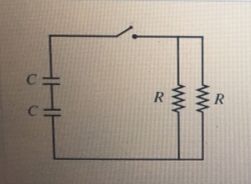# Problem: Suppose that R = 5 kΩ 12 and C = 3 uF.What is the time constant for the discharge of the capacitors in (Figure 1)? Express your answer to two significant figures and include the appropriate units.

###### FREE Expert Solution

The time constant for RC circuits:

$\overline{){\mathbf{\tau }}{\mathbf{=}}{\mathbf{R}}{\mathbf{C}}}$

For two capacitors in series:

$\overline{){{\mathbf{C}}}_{\mathbf{e}\mathbf{q}}{\mathbf{=}}\frac{{\mathbf{C}}_{\mathbf{1}}{\mathbf{C}}_{\mathbf{2}}}{{\mathbf{C}}_{\mathbf{1}}\mathbf{+}{\mathbf{C}}_{\mathbf{2}}}}$

For 2 resistors in parallel:

$\overline{){{\mathbf{R}}}_{{\mathbf{eq}}}{\mathbf{=}}\frac{{\mathbf{R}}_{\mathbf{1}}{\mathbf{R}}_{\mathbf{2}}}{{\mathbf{R}}_{\mathbf{1}}\mathbf{+}{\mathbf{R}}_{\mathbf{2}}}}$

84% (141 ratings)###### Problem Details

Suppose that R = 5 kΩ 12 and C = 3 uF.What is the time constant for the discharge of the capacitors in (Figure 1)?

Express your answer to two significant figures and include the appropriate units.